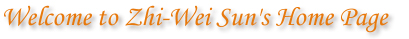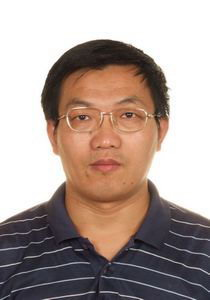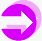visits since 2012

 Zhi-Wei Sun (孙智伟) 　　　　　　Department of Mathematics 　　　　　　Nanjing University 　　　　　　Nanjing 210093 　　　　　　People's Republic of China 　　　　　　E-mail: zwsun@nju.edu.cn 　　　　　　Office in the Dept.: Room 201Last Name Sun First Name Zhi Wei Year of Birth 1965

Research Interests

Number Theory (especially Combinatorial Number Theory),
Combinatorics, Group Theory, Mathematical Logic.

Editorial Board Member of Electronic Research Archive, 2019--.
Reviewer for Zentralblatt Math., 2007--.
Reviewer for Mathematical Reviews, 1992--.
Referee for Adv. in Math., Proc. Amer. Math. Soc., Acta Arith., J. Number Theory, J. Combin. Theory Ser. A, European J. Combin.,
Finite Fields Appl., Adv. in Appl. Math., Discrete Math., Discrete Appl. Math., Ramanujan J., SIAM Review
etc.
I refuse to referee papers for any open access journal which asks for page charges.

School Education and Employment History

1980.9--1983.7 The High Middle School Attached to Nanjing Normal Univ.
1983.9--1992.6 Department of Mathematics, Nanjing University
(Undergraduate--Ph. D. Candidate; B. Sc. 1987, Ph. D. 1992)
1992.7-- 　　　 Teacher in Department of Mathematics, Nanjing University
1994.4--1998.3 Associate Professor in Math.
1998.4-- 　　　 Full Professor in Math.
1999.11- 　　　 Supervisor of Ph. D. students

My paper Further results on Hilbert's Tenth Problem (based on my PhD thesis in 1992)

My book New Conjectures in Number Theory and Combinatorics (数论与组合中的新猜想) (which collects 820 open conjectures posed by me)

My book Modern Algebra (近世代数) （which is a textbook for undergraduate students)

My Favorite Conjecture with $3500 (3500 US dollars) Prize for the First Proof (see OEIS A303389, A303540 and A303821 for similar conjectures) Any integer n > 1 can be written as a2 + b2 + 3c + 5d with a, b, c, d nonnegative integers. [This has been verified for n up to 2.4*1011.] My Four-square Conjecture with$2500 Prize for the First Proof

Every n = 2,3,... can be written as x2 + y2 + (2a3b)2 + (2c5d)2, where x,y,a,b,c,d are nonnegative integers. [This has been verified for n up to 1.6*1011 by Giovanni Resta.]

My 2-4-6-8 Conjecture with $2468 Prize for the First Proof Any positive integer n can be written as binom(w,2) + binom(x,4) + binom(y,6) + binom(z,8) with w,x,y,z integers greater than one. [This has been verified for n up to 2*1012 by Yaakov Baruch.] My 24-Conjecture with$2400 Prize (see also OEIS A281976 and arXiv:1701.05868

Any natural number n can be written as the sum of squares of four nonnegative integers x, y, z and w such that both x and x+24y are squares. [This has been verified for n up to 1010 by Qing-Hu Hou.]

My Conjecture involving Primes and Powers of 2 with $1000 Prize (see also Conjecture 3.6(i) of this paper) Every n = 2, 3, ... can be written as a sum of two positive integers k and m such that 2k + m is prime. [This has been verified for n up to 107.] My Conjecture on Alternating Sums of Consecutive Primes with$1000 Prize (see Conj. 1.3 of this published paper)

For any positive integer m, there are consecutive primes pk,...,pn (k < n) not exceeding 2m+2.2*sqrt(m) such that m = pn - pn-1 + ... + (-1)n-k pk, where pj denotes the j-th prime. [This has been verified for m up to 109 by Chang Zhang.]

My Conjecture on Primitive Roots of the Form x2+1 with 2000 RMB Prize (see OEIS A239957, A241476 and Conj. 3.1 of this paper)

For any prime p, there is an integer 0 < g < p with g-1 an integer square such that g is a primitive root modulo p. [I verified this for all primes below 107. Later, C. Greathouse extended the verification to all primes below 1010.]

My 1680-Conjecture with 1680 RMB Prize (see also OEIS A280831 and Conjecture 4.10(iv) of this published paper

Any natural number n can be written as the sum of squares of four nonnegative integers x, y, z and w such that x4 + 1680y3z is a square. [This has been verified for n up to 108 by Qing-Hu Hou.]

My Conjecture on the Representation n = x4 + y3 + z2 + 2k with $234 Prize (see also Conjecture 6.1(i) of this paper) Each n = 2,3,... can be written as x4 + y3 + z2 + 2k with x,y,z nonnegative integers and k a positive integer. [This has been verified by Qing-Hu Hou for n up to 109.] My Conjecture on Primes of the Form x2+ny2 with$200 Prize (see also Conjecture 2.21(i) of this paper)

Each n = 2,3,... can be written as x+y with x and y positive integers such that x+ny and x2+ny2 are both prime.

My Little 1-3-5 Conjecture with $135 Prize (see this paper for more such conjectures) Each n = 0,1,2,... can be written as x(x+1)/2+y(3y+1)/2+z(5z+1)/2 with x,y,z nonnegative integers. [I have proved the weaker version with x,y,z integers.] My Conjecture related to Bertrand's Postulate with$100 Prize (see also Conjecture 2.18 of this paper)

Let n be any positive integer. Then, for some k=0,...n, both n+k and n+k2 are prime. [I have verified this conjecture for n up to 200,000,000.]

My 100 Conjectures on Representations involving Primes or related Things

My 60 Open Problems on Combinatorial Properties of Primes

My Conjecture on the Prime-Counting Function (see Conjectures 2.1, 2.6 and 2.22 of this paper)

(i) For any integer n>1, π(k*n) is prime for some k = 1,...,n, where π(x) denotes the number of primes not exceeding x. [I have verified this for n up to 107. See OEIS A237578.]
(ii) For every positive integer n, π(π(k*n)) is a square for some k = 1,...,n. [I have verified this for n up to 2*105. See OEIS A238902 and OEIS A239884.]
(iii) For each integer n>2, π(n-p) is a square for some prime p < n. [I have verified this for n up to 5*108. See OEIS A237706 and OEIS A237710.]

My "Super Twin Prime Conjecture" (see Conjecture 3.2 of this paper)

Each n = 3, 4, ... can be written as k + m with k and m positive integers such that p(k) + 2 and p(p(m)) + 2 are both prime, where p(j) denotes the j-th prime. [I have verified this for n up to 109.]

My Conjecture on Unification of Goldbach's Conjecture and the Twin Prime Conjecture (see Conjecture 3.1 of this paper)

Any even number greater than 4 can be written as p + q with p, q and prime(p+2) + 2 all prime, where prime(n) denotes the n-th prime.

My Conjecture involving the n-th Prime (see also Conjecture 4.4 of this paper)

For each m = 1,2,3, ... there is a positive integer n such that m + n divides pm + pn, where pk denotes the k-th prime. Moreover, we may require that n < m*(m-1) if m > 2. [This has been verified for m up to 4*105.]

My Conjecture on Fibonacci Quadratic Nonresidues (see OEIS A241568, OEIS A241604 and A241675)

For each n = 5,6,..., there exists a Fibonacci number F(k) < n/2 such that no square is congruent to F(k) modulo n. [This can be easily reduced to the case with n prime. I have verified it for all primes p with 3 < p < 3*109.]

My Conjecture on Representations involving Cubes (see also Conjecture 1.2(iii) of this paper)

Any positive integer n can be written as the sum of a nonnegative cube, a square and a positive triangular number. [I have verified this for n up to 2*107.]

My Conjecture on Representations involving Fourth Powers (see also Conjecture 1.2(iv) of this paper)

Any nonnegative integer n can be written as x4 + y(3y+1)/2 + z(7z+1)/2 with x,y,z integers.

My Problem Upgrading Waring's Problem (see OEIS A271099, OEIS A271169, A271237, A267826 and A267861)

My 30 Conjectures in Additive Combinatorics

Let G be an additive abelian group of odd order. Then, for any subset A of G with |A|=n>2 there is a numbering a1, ..., an of all the elements of A such that a1+a2, ..., an-1+an, an+a1 are pairwise distinct. [This has been verified for |G|<30 by Yuxuan Ji.]

My Conjectures on Determinants (see also arXiv:1308.2900 and Three mysterious conjectures on Hankel-type determinants)

My 100 Open Conjectures on Congruences （For the old preprint version on arXiv, see arXiv:0911.5665)

My 234 Conjectural Series for Powers of π and Other Constants (2010-2014) and My 117 New Conjectural Series for Powers of π (2019)

Let C(n,k) denote the binomial coefficient n!/(k!(n-k)!) and let Tn(b,c) denote the coefficient of xn in (x2+bx+c)n. Then
k ≥ 0 (126k+31)Tk(22,212)3/(-80)3k = 880*sqrt(5)/(21π),
k ≥ 0 (24k+5)C(2k,k)Tk(4,9)2/282k = 49(sqrt(3)+sqrt(6))/(9π),
k ≥ 0(2800512k+435257)C(2k,k)Tk(73,576)2 /4342k = 10406669/(2sqrt(6)π),
k ≥ 0(40k+13)Tk(4,1)Tk(1,-1)2 /(-50)k = 55*sqrt(15)/(9π),
k ≥ 0(1435k+113)Tk(7,1)Tk(10,10)2 /3240k = 1452*sqrt(5)/π,
k>0(28k2-18k+3)(-64)k /(k5C(2k,k)4C(3k,k)) = -14n>0 1/n3.
n ≥ 0(28n+5)24-2n C(2n,n)k ≥ 0 5k C(2k,k)2C(2(n-k),n-k)2/C(n,k) = 9(sqrt(2)+2)/π.
n ≥ 0 (18n2+7n+1)(-128)-n C(2n,n)2k ≥ 0 C(-1/4,k)2C(-3/4,n-k)2 = 4*sqrt(2)/π2.
n ≥ 0(40n2+26n+5)(-256)-n C(2n,n)2k ≥ 0 C(n,k)2C(2k,k)C(2(n-k),n-k) = 24/π2.

My Conjecture on Recurrence for Primes (see also Conj. 1.2 of this published paper and OEIS A181901)

For any positive integer n different from 1,2,4,9, the (n+1)-th prime pn+1 is just the least positive integer m such that 2sk2 (k=1,...,n) are pairwise distinct modulo m, where sk = pk-pk-1+...+(-1)k-1p1. [This has been verified for n ≤ 300000.]

My Conjecture on Sums of Primes and Triangular Numbers

Each natural number not equal to 216 can be written in the form p+Tx , where p is 0 or a prime, and Tx=x(x+1)/2 is a triangular number. [This has been verified up to 1,000,000,000,000.] In general, for any a,b=0,1,2,... and odd integer r, all sufficiently large integers can be written in the form 2ap +Tx , where p is either zero or a prime congruent to r modulo 2b.

My Conjecture on Sums of Polygonal Numbers

For each integer m>2, any natural number n can be expressed as pm+1(x1) + pm+2(x2) + pm+3(x3) + r with x1,x2,x3 nonnegative integers and r among 0,...,m-3, where pk(x)=(k-2)x(x-1)/2+x (x=0,1,2,...) are k-gonal numbers. In particular, every natural number is the sum of a square, a pentagonal number and a hexagonal number. [For m=3, m=4,...,10, and m=11,...,40, this has been verified for n up to 30,000,000, 500,000 and 100,000 respectively.]

My Conjecture on Disjoint Cosets (see Conjecture 1.2 of this published paper)

Let a1G1 , ..., akGk (k>1) be finitely many pairwise disjoint left cosets in a group G with all the indices [G:Gi] finite. Then, for some distinct i and j the greatest common divisor of [G:Gi] and [G:Gj] is at least k.

My Conjecture on Covers of Groups

Let a1G1 , ..., akGk be finitely many left cosets in a group G which cover all the elements of G at least m>0 times with ajGj irredundant. Then k is at least m+f([G:Gj]), where f(1)=0 and f(p1 ... pr) =(p1-1) + ... +(pr-1) for any primes p1 , ..., pr .

My Conjecture on Linear Extension of the Erdos-Heilbronn Conjecture

Redmond-Sun Conjecture (in PlanetMath.)

Publications

Papers Indexed in SCI or SCI-E
Recent Publications (2008-) Preprints on arXiv
Publications during 2000-2007 Publications during 1987-1999

Other Information

Research Grants Awards and Honours
Academic Visits Courses Taught and Ph.D Students
Notes on Some Conjectures of Z. W. Sun Introduction to Sun's Papers on Covers
Books and Papers Citing Sun's Work Webpages of Wall-Sun-Sun Prime [1, 2, 3]
Covers, Sumsets and Zero-sums Link to the useful Number Theory Web
Mixed Sums of Primes and Other Terms Articles on arXiv: Combinatorics, Number Theory

My Mathematical Lectures or Talks

Selected Photographs
1. at Venice (Venezia), Florence (Firenze), Genova, Rome (Roma), Trieste (2004)
2. at Vienna (Wien), Graz (2004), Lyon, Bordeaux, Pacific beach (2005), Osaka (2008)
3. at MIT [1,2] and University of California at Irvine [1,2,3] (2006)
4. at Univ. of Wisconsin at Madison and Univ. of Illinois at Urbana Champaign (2006)
5. at Stanford University [1, 2, 3, 4, 5] (2010)
6. with Prof. M. Agrawal (famous for the AKS primality test) at ICTP, Trieste (2004)
7. with Prof. R. Schoof (famous for Schoof's algorithm) at Rome (2004)
8. with Prof. A. Perelli at Genova (2004)
9. with Prof. A. Geroldinger and his wife at Graz (2004)
10. with Prof. R. P. Stanley, R. A. Askey and J. Zeng at Tianjin (2004)
11. with Prof. Y. Bilu at Bordeaux (2005)
12. with Prof. J. Zeng and Prof. J. L. Nicolas at Institute of Camille Jordan (2005)
13. Photographs at the Integers Conference 2005 (West Georgia Univ., Oct. 27-30):
Prof. R. L. Graham, the Graham couple, Prof. C. Pomerance,
Prof. M. B. Nathanson, Prof. Fan Chung and M. B. Nathanson, Prof. B. Landman;
with Prof. R.L. Graham and Fan Chung, C. Pomerance and S. Wagstaff,
with Prof. M. B. Nathanson, H. Diamond and D. Goldston, B. Landman, S. Milne,
with Prof. A. Bialostocki, T. Brown, K. O'Bryant and R. L. Jin, V. F. Lev.
14. with Prof. R. Askey, K. Ono and T. H. Yang at Univ. of Wisconsin at Madison (2006)
15. with Prof. H. Halberstam and P. T. Bateman at UI Urbana-Champaign (2006)
16. with Prof. R. P. Stanley at MIT (Massachusetts Institute of Technology) (2006),
with Prof. B. Green (famous for the Green-Tao theorem) at his MIT office (2006)
17. with Prof. K. Rubin and D. Wan at Univ. of California at Irvine (2006)
18. with Prof. B. Berndt at Nanjing University (2006)
19. with Prof. A. Schinzel at Weihai (Shandong Province, China) (2006)
20. with Prof. W. Konen at Osaka (Japan) (2008)
21. with Prof. R. Schoof and Z. H. Sun in Xuanwu Park at Nanjing (2008)
22. with Prof. M. Waldschmidt in India (2010)
23. with Prof. S. D. Adhikari and Dr. D. J. Grynkiewicz in India (2010)
24. with Prof. Wen-Ching Li, Don Zagier and his wife in Taiwan (2010)
25. Pictures at the 1st Workshop on Number Theory, Combinatorics and their Interactions (Nanjing Univ., August 10-12, 2007):
A Collective Photograph of Participants, Prof. Wen-Ching Winnie Li [1, 2, 3];
Prof. Qin Yue, Zhi-Wei Sun, Keqin Feng, Wen-Ching W. Li, Daqing Wan and Yong-Gao Chen;
W. C. Li, Z. W. Sun and D. Wan, W. C. Li and Z. W. Sun, W. C. Li and D. Wan.Zhi-Wei Sun has the copyright of those unpublished materials at this website.
The copyright of each published or accepted paper is held by the corresponding publisher.